# Quiz 21: Real Estate Investment Trusts Reits

Business

The financial statements of the trust have been given in the problem. The financial ratio which would be required for the analysis is the earning per share (EPS), Net operating income (NOI), funds from operation (FFO), Return on Assets (ROA). (a) Assuming the dividends are 95% of the EPS, the dividends would be,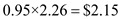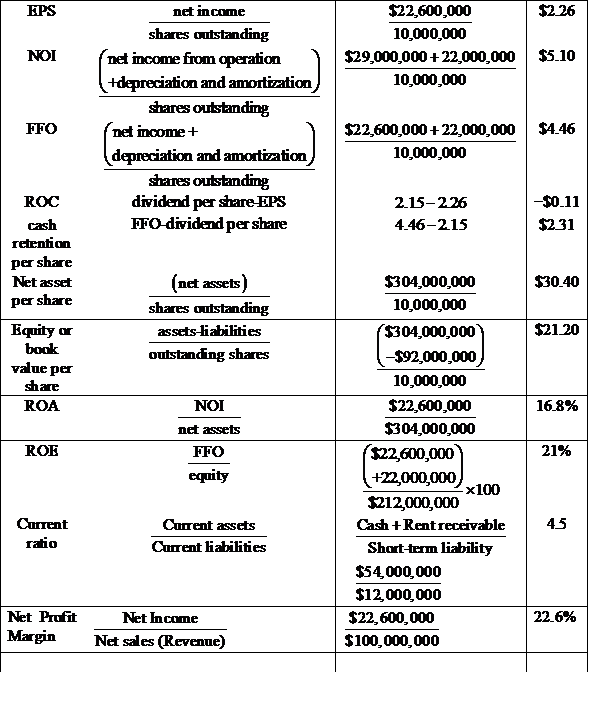(b) From the above calculation the dividend which the company pays is \$2.15 per share and the dividend yield is 8%. Hence, the indicated price would become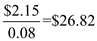The price range for the company is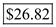Given, that the market research indicates that investors Pay (12 x FFO) for comparable REITs. Hence in this case the price would be now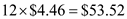The price range is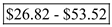(c) The net asset value is calculated using the formula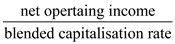Given: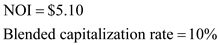Substituting values in the above equation the NAV is calculated as follows: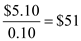Hence, the NAV with the blended capitalization of 10% is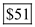A real estate investment trust (REIT) is a trust that has been elected to qualify under certain tax provisions. It is a pass-through entity that distributes to its shareholders all of its taxable earnings in addition to any capital gains generated from the sale of properties. To comply and to maintain the tax exempt status, real estate investment trusts (REITs) must fulfill certain general requirements as: 1. At least 95% of the gross income of REIT must be from dividends, interests, rent or capital gains from the sale of the assets. 2. At least 75% of the gross income should be from the rent, interests on obligations backed up by mortgages, capital gains from the sale of assets or income from investments in other REITs. 3. 75% of the value of the REIT assets must be from real estate assets, cash and government securities. 4. A REIT should distribute 95% of its taxable income as dividend to shareholders.

Earnings-per-share (EPS) is the percentage or a portion of the profits generated by a firm that are allocated to each of outstanding share of common stock. Funds-from-Operations (FFO) is a measure used in real estate business to determine the cash flow from the operating activities. It is calculated by adding the values of depreciation, amortization amounts to the earnings. Return on capital (ROC) is a ratio used to compare the profitability and value creating capacity of various firms basing on the amount of capital invested in a company. Approach 1 Prepare the statement of balance sheet.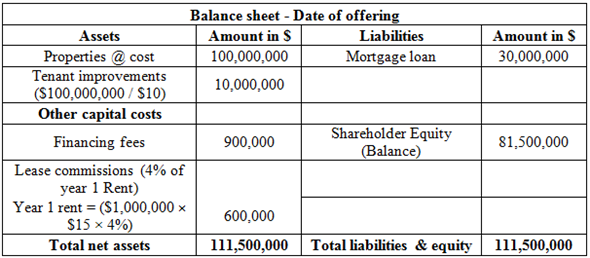Prepare the operating statement.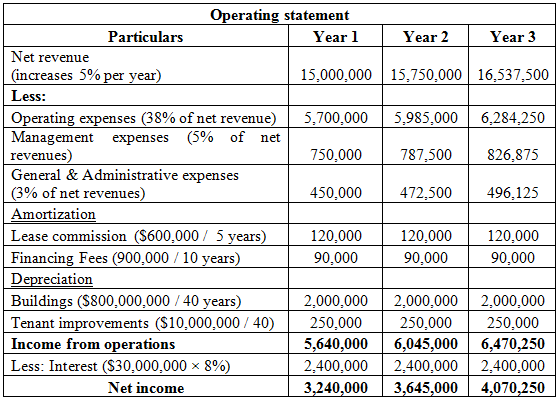Calculate the relevant ratios of year 1.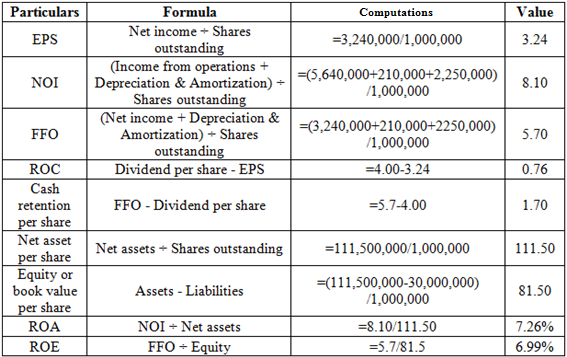Therefore, under approach 1, EPS is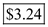, FFO is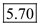and the ROC is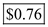. Approach 2 Prepare statement of balance sheet.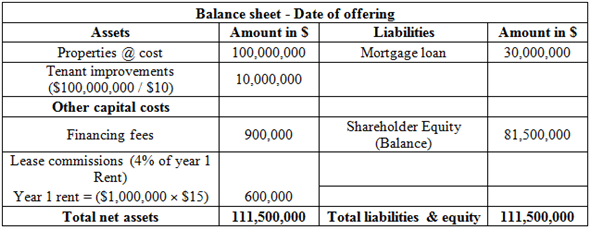Prepare operating statement.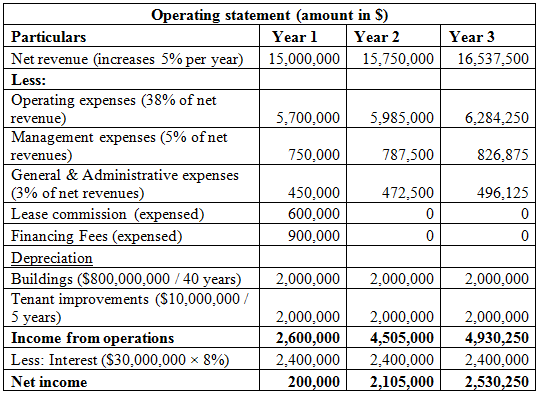Calculate the relevant ratios of year 1.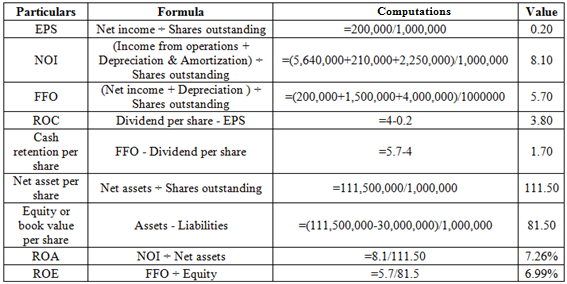Therefore, under approach 2, EPS is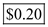, FFO is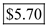and the ROC is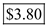. Compare the ratios under both the approaches particularly EPS and ROC.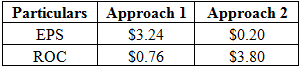It is evident from the above results that the EPS under approach 1 is higher with lower ROC. As a result if \$4.00 dividend per share is paid, shareholders will have to face higher taxation. FFO per share, the main focus of the investors is \$5.70 under both the approaches. So, the company has to adopt the accounting treatment carefully.